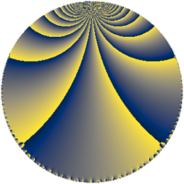# Properties

 Label 2304.3.g.jLevel $2304$ Weight $3$ Character orbit 2304.g Analytic conductor $62.779$ Analytic rank $0$ Dimension $2$ CM discriminant -8 Inner twists $4$

# Related objects

## Newspace parameters

 Level: $$N$$ $$=$$ $$2304 = 2^{8} \cdot 3^{2}$$ Weight: $$k$$ $$=$$ $$3$$ Character orbit: $$[\chi]$$ $$=$$ 2304.g (of order $$2$$, degree $$1$$, not minimal)

## Newform invariants

 Self dual: no Analytic conductor: $$62.7794529086$$ Analytic rank: $$0$$ Dimension: $$2$$ Coefficient field: $$\Q(\sqrt{-1})$$ Defining polynomial: $$x^{2} + 1$$ Coefficient ring: $$\Z[a_1, \ldots, a_{19}]$$ Coefficient ring index: $$2$$ Twist minimal: no (minimal twist has level 8) Sato-Tate group: $\mathrm{U}(1)[D_{2}]$

## $q$-expansion

Coefficients of the $$q$$-expansion are expressed in terms of $$i = \sqrt{-1}$$. We also show the integral $$q$$-expansion of the trace form.

 $$f(q)$$ $$=$$ $$q +O(q^{10})$$ $$q -14 i q^{11} -2 q^{17} + 34 i q^{19} -25 q^{25} -46 q^{41} + 14 i q^{43} + 49 q^{49} + 82 i q^{59} -62 i q^{67} + 142 q^{73} + 158 i q^{83} + 146 q^{89} -94 q^{97} +O(q^{100})$$ $$\operatorname{Tr}(f)(q)$$ $$=$$ $$2q + O(q^{10})$$ $$2q - 4q^{17} - 50q^{25} - 92q^{41} + 98q^{49} + 284q^{73} + 292q^{89} - 188q^{97} + O(q^{100})$$

## Character values

We give the values of $$\chi$$ on generators for $$\left(\mathbb{Z}/2304\mathbb{Z}\right)^\times$$.

 $$n$$ $$1279$$ $$1793$$ $$2053$$ $$\chi(n)$$ $$-1$$ $$1$$ $$1$$

## Embeddings

For each embedding $$\iota_m$$ of the coefficient field, the values $$\iota_m(a_n)$$ are shown below.

For more information on an embedded modular form you can click on its label.

Label $$\iota_m(\nu)$$ $$a_{2}$$ $$a_{3}$$ $$a_{4}$$ $$a_{5}$$ $$a_{6}$$ $$a_{7}$$ $$a_{8}$$ $$a_{9}$$ $$a_{10}$$
1279.1
 1.00000i − 1.00000i
0 0 0 0 0 0 0 0 0
1279.2 0 0 0 0 0 0 0 0 0
 $$n$$: e.g. 2-40 or 990-1000 Significant digits: Format: Complex embeddings Normalized embeddings Satake parameters Satake angles

## Inner twists

Char Parity Ord Mult Type
1.a even 1 1 trivial
8.d odd 2 1 CM by $$\Q(\sqrt{-2})$$
4.b odd 2 1 inner
8.b even 2 1 inner

## Twists

By twisting character orbit
Char Parity Ord Mult Type Twist Min Dim
1.a even 1 1 trivial 2304.3.g.j 2
3.b odd 2 1 256.3.c.e 2
4.b odd 2 1 inner 2304.3.g.j 2
8.b even 2 1 inner 2304.3.g.j 2
8.d odd 2 1 CM 2304.3.g.j 2
12.b even 2 1 256.3.c.e 2
16.e even 4 1 72.3.b.a 1
16.e even 4 1 288.3.b.a 1
16.f odd 4 1 72.3.b.a 1
16.f odd 4 1 288.3.b.a 1
24.f even 2 1 256.3.c.e 2
24.h odd 2 1 256.3.c.e 2
48.i odd 4 1 8.3.d.a 1
48.i odd 4 1 32.3.d.a 1
48.k even 4 1 8.3.d.a 1
48.k even 4 1 32.3.d.a 1
240.t even 4 1 200.3.g.a 1
240.t even 4 1 800.3.g.a 1
240.z odd 4 1 200.3.e.a 2
240.z odd 4 1 800.3.e.a 2
240.bb even 4 1 200.3.e.a 2
240.bb even 4 1 800.3.e.a 2
240.bd odd 4 1 200.3.e.a 2
240.bd odd 4 1 800.3.e.a 2
240.bf even 4 1 200.3.e.a 2
240.bf even 4 1 800.3.e.a 2
240.bm odd 4 1 200.3.g.a 1
240.bm odd 4 1 800.3.g.a 1
336.v odd 4 1 392.3.g.a 1
336.v odd 4 1 1568.3.g.a 1
336.y even 4 1 392.3.g.a 1
336.y even 4 1 1568.3.g.a 1
336.bo even 12 2 392.3.k.b 2
336.br odd 12 2 392.3.k.b 2
336.bt odd 12 2 392.3.k.d 2
336.bu even 12 2 392.3.k.d 2

By twisted newform orbit
Twist Min Dim Char Parity Ord Mult Type
8.3.d.a 1 48.i odd 4 1
8.3.d.a 1 48.k even 4 1
32.3.d.a 1 48.i odd 4 1
32.3.d.a 1 48.k even 4 1
72.3.b.a 1 16.e even 4 1
72.3.b.a 1 16.f odd 4 1
200.3.e.a 2 240.z odd 4 1
200.3.e.a 2 240.bb even 4 1
200.3.e.a 2 240.bd odd 4 1
200.3.e.a 2 240.bf even 4 1
200.3.g.a 1 240.t even 4 1
200.3.g.a 1 240.bm odd 4 1
256.3.c.e 2 3.b odd 2 1
256.3.c.e 2 12.b even 2 1
256.3.c.e 2 24.f even 2 1
256.3.c.e 2 24.h odd 2 1
288.3.b.a 1 16.e even 4 1
288.3.b.a 1 16.f odd 4 1
392.3.g.a 1 336.v odd 4 1
392.3.g.a 1 336.y even 4 1
392.3.k.b 2 336.bo even 12 2
392.3.k.b 2 336.br odd 12 2
392.3.k.d 2 336.bt odd 12 2
392.3.k.d 2 336.bu even 12 2
800.3.e.a 2 240.z odd 4 1
800.3.e.a 2 240.bb even 4 1
800.3.e.a 2 240.bd odd 4 1
800.3.e.a 2 240.bf even 4 1
800.3.g.a 1 240.t even 4 1
800.3.g.a 1 240.bm odd 4 1
1568.3.g.a 1 336.v odd 4 1
1568.3.g.a 1 336.y even 4 1
2304.3.g.j 2 1.a even 1 1 trivial
2304.3.g.j 2 4.b odd 2 1 inner
2304.3.g.j 2 8.b even 2 1 inner
2304.3.g.j 2 8.d odd 2 1 CM

## Hecke kernels

This newform subspace can be constructed as the intersection of the kernels of the following linear operators acting on $$S_{3}^{\mathrm{new}}(2304, [\chi])$$:

 $$T_{5}$$ $$T_{7}$$ $$T_{11}^{2} + 196$$ $$T_{13}$$

## Hecke characteristic polynomials

$p$ $F_p(T)$
$2$ $$T^{2}$$
$3$ $$T^{2}$$
$5$ $$T^{2}$$
$7$ $$T^{2}$$
$11$ $$196 + T^{2}$$
$13$ $$T^{2}$$
$17$ $$( 2 + T )^{2}$$
$19$ $$1156 + T^{2}$$
$23$ $$T^{2}$$
$29$ $$T^{2}$$
$31$ $$T^{2}$$
$37$ $$T^{2}$$
$41$ $$( 46 + T )^{2}$$
$43$ $$196 + T^{2}$$
$47$ $$T^{2}$$
$53$ $$T^{2}$$
$59$ $$6724 + T^{2}$$
$61$ $$T^{2}$$
$67$ $$3844 + T^{2}$$
$71$ $$T^{2}$$
$73$ $$( -142 + T )^{2}$$
$79$ $$T^{2}$$
$83$ $$24964 + T^{2}$$
$89$ $$( -146 + T )^{2}$$
$97$ $$( 94 + T )^{2}$$